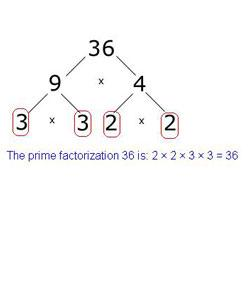# Interesting Quiz Over Prime Factorization

5 Questions | Total Attempts: 304SettingsDo you still remember how to do prime factoring? Then take this quiz to see how good you are!

• 1.
What are the prime factors of 16?
• A.

2 x 2 x 2 x 2

• B.

2 x 8

• C.

2 x 2 x 4

• 2.
The prime factors of 18 is 2 x 3 x 3.
• A.

True

• B.

False

• 3.
What are the different ways that you can use prime factorization? List all the choices that are true.
• A.

• B.

The factor tree.

• C.

Multiplying

• 4.
2 x       x 3 = 12 What number is in the blank?
• 5.
How do you do prime factorization? List all the steps.
Related TopicsBack to top